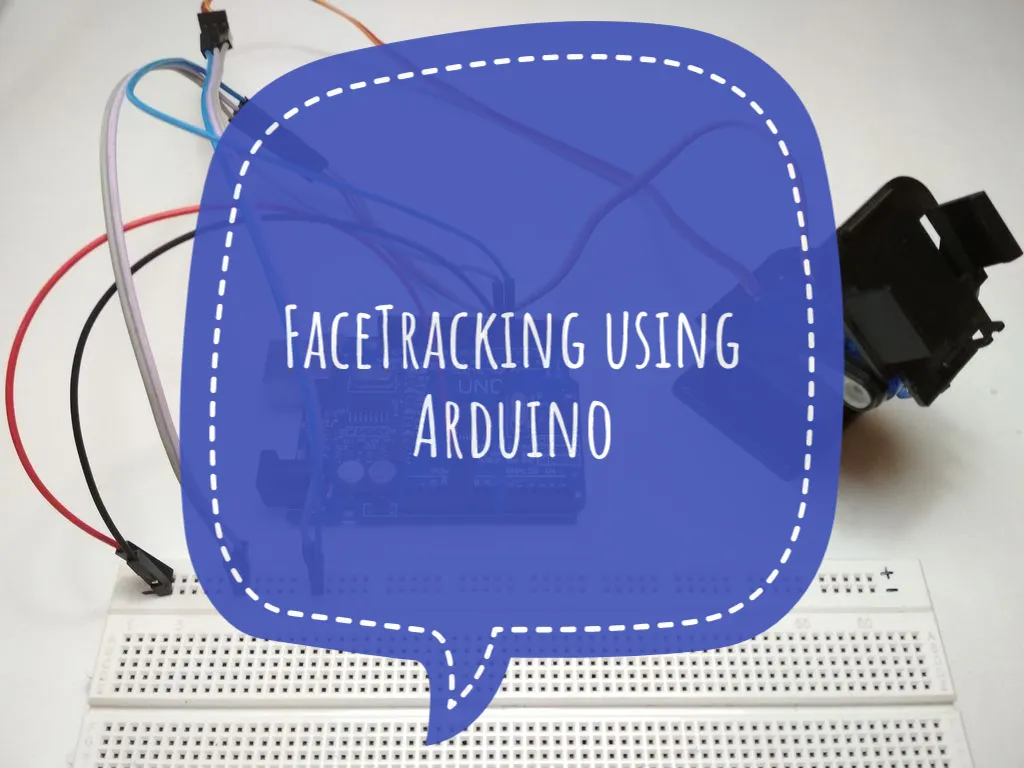# Face Tracking Using Arduino

Python does the image processing, Arduino controls the servos.## Things used in this project

### Hardware componentsArduino UNO & Genuino UNO
×1
 web cam
×1
 Micro servos
×2
 Pan-Tilt
×1×1

### Software apps and online services

 Python 2.7OpenCV

## Code

### servo.ino

Arduino
Error opening file.

### Code snippet #2

Plain text
```#import all the required modules

import numpy as np
import serial
import time
import sys
import cv2

#Setup Communication path for arduino (In place of 'COM5' put the port to which your arduino is connected)
arduino = serial.Serial('COM5', 9600)
time.sleep(2)
print("Connected to arduino...")

#importing the Haarcascade for face detection

#To capture the video stream from webcam.
cap = cv2.VideoCapture(0)

#Read the captured image, convert it to Gray image and find faces
while 1:
cv2.resizeWindow('img', 500,500)
cv2.line(img,(500,250),(0,250),(0,255,0),1)
cv2.line(img,(250,0),(250,500),(0,255,0),1)
cv2.circle(img, (250, 250), 5, (255, 255, 255), -1)
gray  = cv2.cvtColor(img, cv2.COLOR_BGR2GRAY)

#detect the face and make a rectangle around it.
for (x,y,w,h) in faces:
cv2.rectangle(img,(x,y),(x+w,y+h),(0,255,0),5)
roi_gray  = gray[y:y+h, x:x+w]
roi_color = img[y:y+h, x:x+w]

arr = {y:y+h, x:x+w}
print (arr)

print ('X :' +str(x))
print ('Y :'+str(y))
print ('x+w :' +str(x+w))
print ('y+h :' +str(y+h))

# Center of roi (Rectangle)
xx = int(x+(x+h))/2
yy = int(y+(y+w))/2
print (xx)
print (yy)
center = (xx,yy)

# sending data to arduino
print("Center of Rectangle is :", center)
data = "X{0:d}Y{1:d}Z".format(xx, yy)
print ("output = '" +data+ "'")
arduino.write(data)

#Display the stream.
cv2.imshow('img',img)

#Hit 'Esc' to terminate execution
k = cv2.waitKey(30) & 0xff
if k == 27:
break
```

### Code snippet #3

Plain text
```#include<servo.h>

Servo servoVer; //Vertical Servo
Servo servoHor; //Horizontal Servo

int x;
int y;

int prevX;
int prevY;

void setup()
{
Serial.begin(9600);
servoVer.attach(5); //Attach Vertical Servo to Pin 5
servoHor.attach(6); //Attach Horizontal Servo to Pin 6
servoVer.write(90);
servoHor.write(90);
}

void Pos()
{
if(prevX != x || prevY != y)
{
int servoX = map(x, 600, 0, 70, 179);
int servoY = map(y, 450, 0, 179, 95);

servoX = min(servoX, 179);
servoX = max(servoX, 70);
servoY = min(servoY, 179);
servoY = max(servoY, 95);

servoHor.write(servoX);
servoVer.write(servoY);
}
}

void loop()
{
if(Serial.available() > 0)
{
{
x = Serial.parseInt();
{
y = Serial.parseInt();
Pos();
}
}
while(Serial.available() > 0)
{